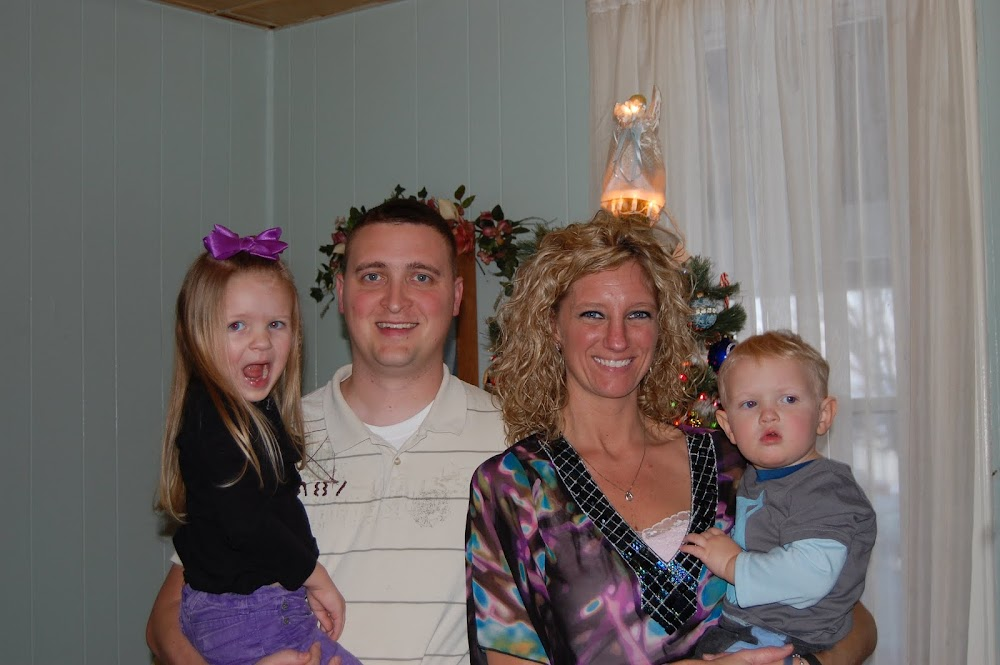## Thursday, December 16, 2010

### Details

Baby Hall #3 has a due date of July 7, 2011. Sound Familiar? It should! It's MIA'S BIRTHDAY!! How did we plan that? Very carefully ;) Haha, as it turns out, we didn't plan it. It's just fate!
Based on my last 2 pregnancies/deliveries--I'm guessing this baby will come in late June. I tend to go a couple of weeks early, and still have healthy sized babes :)
^^^^^^^^^^^^^^^^^^^^^^^^^^^^^^^^^^^^^^^^^^^^^^^^^^^^^^^^^^^^^
I'm 11 weeks, have gained 2 lbs, and can still button all of my pants :)
So far, so good!
^^^^^^^^^^^^^^^^^^^^^^^^^^^^^^^^^^^^^^^^^^^^^^^^^^^^^^^^^^^^^^^^
I'll post a belly pic when it decided to pop...give it a couple more weeks!
^^^^^^^^^^^^^^^^^^^^^^^^^^^^^^^^^^^^^^^^^^^^^^^^^^^^^^^^^^^^^^
Thanks for all of the congrats!